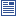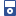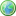Accessibility options:

# Simultaneous linear equations resources

Show me all resources applicable to

### Community Project (1)Differentiation for Economics and Business Studies Functions of Multi-Variable Functions (SOURCE)
Latex source, image files and metadata for the Fact & Formulae leaflet "Differentiation for Economics and Business Studies Functions of Multi-Variable Functions" contributed to the mathcentre Community Project by Morgiane Richard (University of Aberdeen) and reviewed by Anthony Cronin (University College Dublin).

### Guides (1)Just the Maths (A.J.Hobson)
"Just the Maths" authored by the late Tony Hobson, former Senior Lecturer in Mathematics of the School of Mathematical and Information Sciences at Coventry University, is a collection of separate mathematics units, in chronological topic-order, intended for foundation level and first year degree level in higher education where mathematics is a service discipline e.g. engineering.

### iPOD Video (7)Simultaneous Linear Equations Part 1
IPOD VIDEO: The purpose of this section is to look at the solution of simultaneous linear equations. We will see that solving a pair of simultaneous equations is equivalent to finding the location of the point of intersection of two straight lines. This resource is released under a Creative Commons license Attribution-Non-Commercial-No Derivative Works and the copyright is held by Skillbank Solutions Ltd.Simultaneous Linear Equations Part 2
IPOD VIDEO: The purpose of this section is to look at the solution of simultaneous linear equations. We will see that solving a pair of simultaneous equations is equivalent to finding the location of the point of intersection of two straight lines. This resource is released under a Creative Commons license Attribution-Non-Commercial-No Derivative Works and the copyright is held by Skillbank Solutions Ltd.Simultaneous Linear Equations Part 3
IPOD VIDEO: The purpose of this section is to look at the solution of simultaneous linear equations. We will see that solving a pair of simultaneous equations is equivalent to finding the location of the point of intersection of two straight lines. This resource is released under a Creative Commons license Attribution-Non-Commercial-No Derivative Works and the copyright is held by Skillbank Solutions Ltd.Simultaneous Linear Equations Part 4
IPOD VIDEO: The purpose of this section is to look at the solution of simultaneous linear equations. We will see that solving a pair of simultaneous equations is equivalent to finding the location of the point of intersection of two straight lines. This resource is released under a Creative Commons license Attribution-Non-Commercial-No Derivative Works and the copyright is held by Skillbank Solutions Ltd.Simultaneous Linear Equations Part 5
IPOD VIDEO: The purpose of this section is to look at the solution of simultaneous linear equations. We will see that solving a pair of simultaneous equations is equivalent to finding the location of the point of intersection of two straight lines. This resource is released under a Creative Commons license Attribution-Non-Commercial-No Derivative Works and the copyright is held by Skillbank Solutions Ltd.Simultaneous Linear Equations Part 6
IPOD VIDEO: The purpose of this section is to look at the solution of simultaneous linear equations. We will see that solving a pair of simultaneous equations is equivalent to finding the location of the point of intersection of two straight lines. This resource is released under a Creative Commons license Attribution-Non-Commercial-No Derivative Works and the copyright is held by Skillbank Solutions Ltd.Simultaneous Linear Equations Part 7
IPOD VIDEO: The purpose of this section is to look at the solution of simultaneous linear equations. We will see that solving a pair of simultaneous equations is equivalent to finding the location of the point of intersection of two straight lines. This resource is released under a Creative Commons license Attribution-Non-Commercial-No Derivative Works and the copyright is held by Skillbank Solutions Ltd.

### Quick Reference (2)Simultaneous equations
On occasions you will come across two or more unknown quantities, and two or more equations relating to them. These are called simultaneous equations and when asked to solve them yo umust find values of the unknowns which satisfy all the given equations at the same time. On this leaflet we will illustrate one way in which this can be done.Simultaneous equations
This leaflet explains how two equations in two unknowns can be solved by elimination. (Engineering Maths First Aid Kit 2.13)

### Teach Yourself (1)Simultaneous linear equations
This is a complete workbook introducing the solution of a pair of simultaneous linear equations. It contains plenty of examples and exercises. It can be used as a free-standing resource or in conjunction with the mathtutor DVD.

### Test Yourself (4)Maths EG
Computer-aided assessment of maths, stats and numeracy from GCSE to undergraduate level 2. These resources have been made available under a Creative Common licence by Martin Greenhow and Abdulrahman Kamavi, Brunel University.Simultaneous equations - Numbas
Two questions on solving systems of simultaneous equations. Numbas resources have been made available under a Creative Commons licence by Bill Foster and Christian Perfect, School of Mathematics & Statistics at Newcastle University.System of linear equations - Numbas
3 questions. First, two equations in two unknowns, second 3 equations in 3 unknowns, solved by Gauss elimination. The third two equations in 2 unknowns solved by putting into matrix form and finding the inverse of the coefficient matrix. Numbas resources have been made available under a Creative Commons licence by the School of Mathematics & Statistics at Newcastle University.Systems of linear equations - Numbas
3 questions. First, two equations in two unknowns; second 3 equations in 3 unknowns, solved by Gauss elimination. The third, two equations in 2 unknowns solved by putting into matrix form and finding the inverse of the coefficient matrix. Numbas resources have been made available under a Creative Commons licence by Bill Foster and Christian Perfect, School of Mathematics & Statistics at Newcastle University.

### Third Party Resources (2)Mathematics Support Materials from the University of Plymouth
Support material from the University of Plymouth:
The output from this project is a library of portable, interactive, web based support packages to help students learn various mathematical ideas and techniques and to support classroom teaching.
There are support materials on ALGEBRA, GRAPHS, CALCULUS, and much more.
This material is offered through the mathcentre site courtesy of Dr Martin Lavelle and Dr Robin Horan from the University of Plymouth.University of East Anglia (UEA) Interactive Mathematics and Statistics Resources
The Learning Enhancement Team at the University of East Anglia (UEA) has developed la series of interactive resources accessible via Prezi mind maps : Steps into Numeracy, Steps into Algebra, Steps into Trigonometry, Bridging between Algebra and Calculus, Steps into Calculus, Steps into Differential Equations, Steps into Statistics and Other Essential Skills.

### Video (2)Simultaneous linear equations - an Animation
This mathtutor animation shows how solutions to simultaneous linear equations may be found. This resource is released under a Creative Commons license Attribution-Non-Commercial-No Derivative Works and the copyright is held by Skillbank Solutions Ltd.Simultaneous Linear Equations Part 1
The purpose of this section is to look at the solution of simultaneous linear equations. We will see that solving a pair of simultaneous equations is equivalent to finding the location of the point of intersection of two straight lines. (Mathtutor Video Tutorial) This resource is released under a Creative Commons license Attribution-Non-Commercial-No Derivative Works and the copyright is held by Skillbank Solutions Ltd.

### Video with captions which require edits (1)Simultaneous Linear Equations Part 1
The purpose of this section is to look at the solution of simultaneous linear equations. We will see that solving a pair of simultaneous equations is equivalent to finding the location of the point of intersection of two straight lines. (Mathtutor Video Tutorial) The video is released under a Creative Commons license Attribution-Non-Commercial-No Derivative Works and the copyright is held by Skillbank Solutions Ltd.

Website design by Pink Mayhem, Leicester